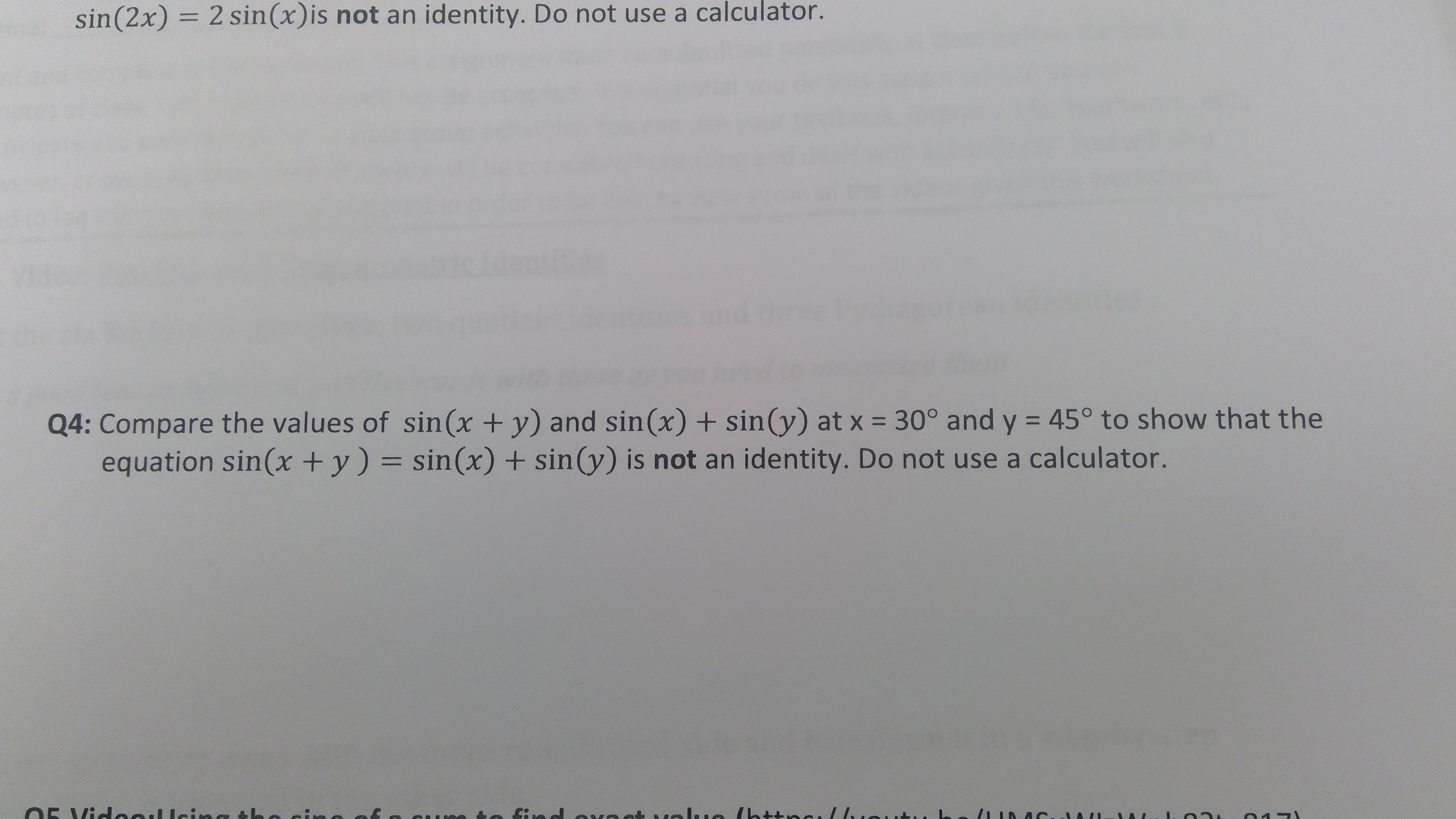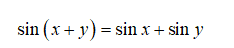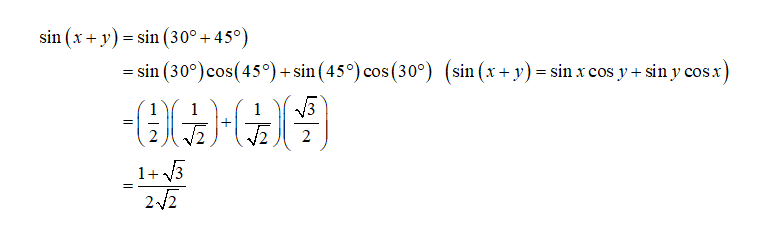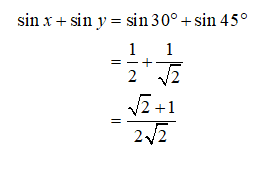# sin(2x) = 2 sin(x)is not an identity. Do not use a calculator.Q4: Compare the values of sin(x +y) and sin(x) + sin(y) at x = 30° and y = 45° to show that theequation sin(x + y) = sin(x) + sin(y) is not an identity. Do not use a calculator.O5 Vie eelleing the cine o fe cunete fin d evect value /http //ueut.

Question
24 viewshelp_outlineImage Transcriptionclosesin(2x) = 2 sin(x)is not an identity. Do not use a calculator. Q4: Compare the values of sin(x +y) and sin(x) + sin(y) at x = 30° and y = 45° to show that the equation sin(x + y) = sin(x) + sin(y) is not an identity. Do not use a calculator. O5 Vie eelleing the cine o fe cunete fin d evect value /http //ueut. fullscreen
check_circle

Step 1

The trigonometric equation to be proved is given as,Step 2

Here x=30o and y=45o.

LHSStep 3

RHS...

### Want to see the full answer?

See Solution

#### Want to see this answer and more?

Solutions are written by subject experts who are available 24/7. Questions are typically answered within 1 hour.*

See Solution
*Response times may vary by subject and question.
Tagged in